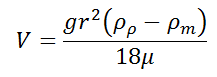# Terminal Velocity using Stoke's Law Calculator

This CalcTown calculator calculates the terminal velocity of a spherical object through an incompressible liquid with low viscosity.

Kg/m3
Kg/m3
m
N-s/m2

#### Result

m/swhere

ρp: Particle Density, in Kg/m^3
ρm: Medium Density, in Kg/m^3
r: Particle Diameter, in meter
V: Fall or Settling Velocity, in meter/second
μ: Medium Viscosity, in N.s/m^2